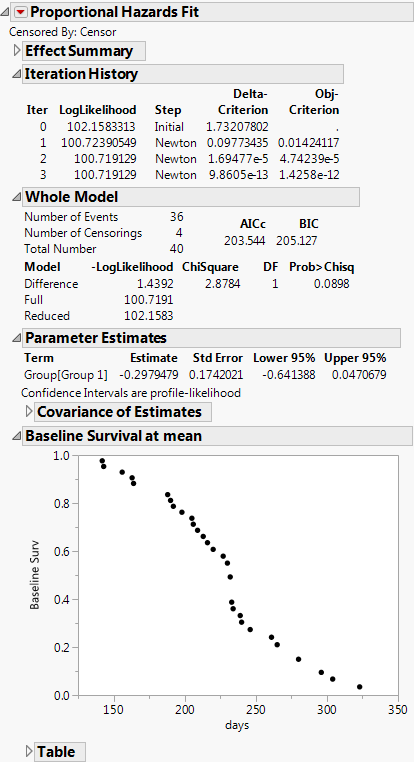Reliability and Survival Methods > Fit Proportional Hazards
Publication date: 07/30/2020

# Fit Proportional Hazards

##### Fit Survival Data Using Semiparametric Regression Models

The Fit Proportional Hazards platform fits the Cox proportional hazards model, which assumes a multiplying relationship between covariates (predictors) and the hazard function.

Proportional hazards models are popular regression models for survival data with covariates. This model is semiparametric. The linear model is estimated, but the form of the hazard function is not. Time-varying covariates are not supported.

Note: The Fit Proportional Hazards platform is a slightly customized version of the Fit Model platform.

Figure 15.1 Example of a Proportional Hazards Fit